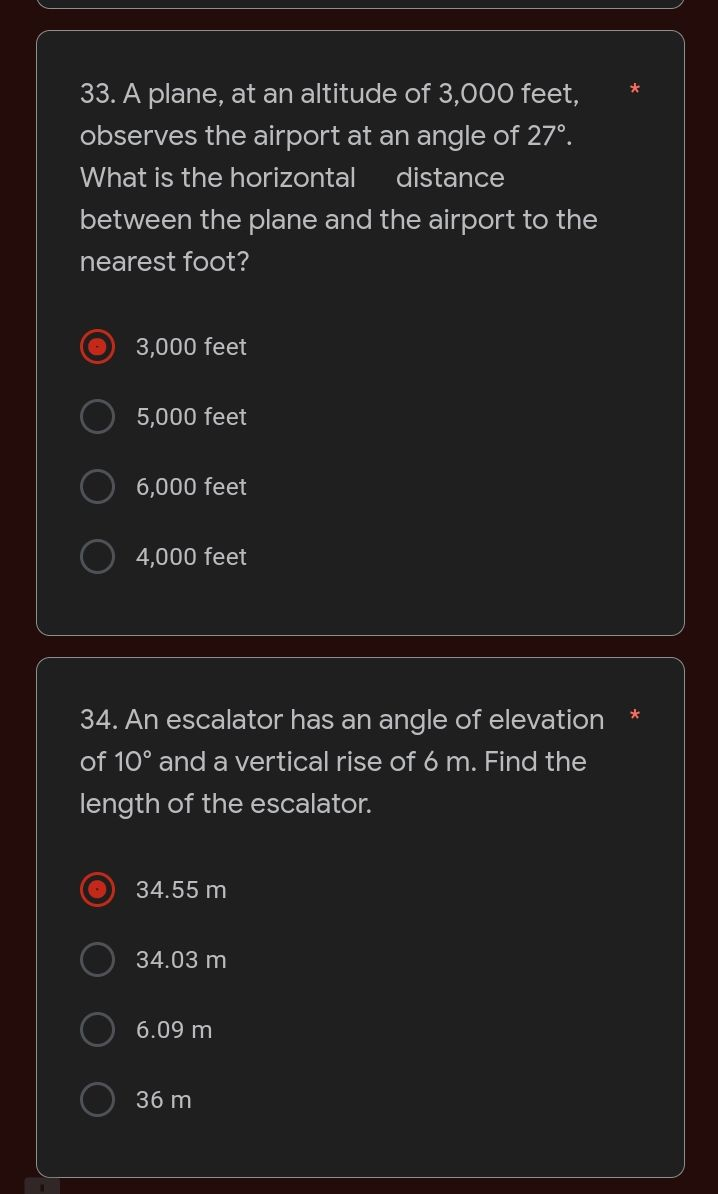### Still have math questions?

Trigonometry
QuestionA plane, at an altitude of $$3,000$$ feet, observes the airport at an angle of $$27 ^ { \circ }$$ . What is the horizontal distance between the plane and the airport to the nearest foot?

$$3,000$$ feet

$$5,000$$ feet

$$6,000$$ feet

$$4,000$$ feet

34. An escalator has an angle of elevation * of $$10 ^ { \circ }$$ and a vertical rise of $$6 m$$ . Find the length of the escalator.

$$34.55 m$$

$$34.03 m$$

$$6.09 m$$

$$36 m$$

1. $$6,000$$ feet
2. $$34.55 m$$